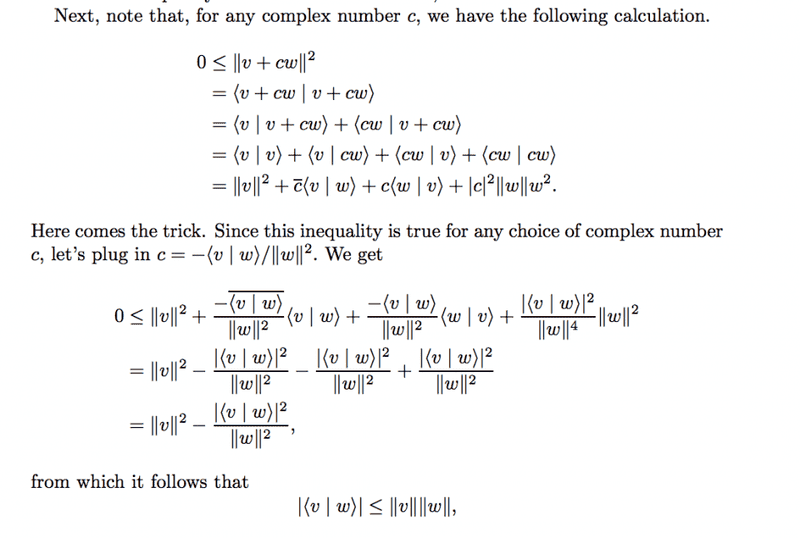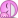# Cauchy-Schwartz Inequality Proof

Gold Member

## Homework Statement

Show that |<v|w>|^2 ≤ <v|v><w|w>
for any |v>,|w> ∈ ℂ^2

## The Attempt at a Solution

The Cauchy-Schwartz inequality is extremely relevant for the math/physics that I am interested in.
I feel like I have a very good proof here, but I am interested in a few things here.
1) Is my proof correct in the right context (Complex numbers here)
2) What is your favorite proof of the Cauchy Schwartz Inequality? (I want to master this one)

Proof:
Case 1: We have to show that if |v> or |w> = 0, then the sides are equal to 0. Which is somewhat trivial to prove by simply plugging in the values and reaching the conclusion using inner space axioms.

Case 2: Where |v> and |w> are not equal to 0.
Here, I let:
a = <v|v>
b = 2<v|w>
c = <w|w>

By Inner product axiom 3 stating that the inner product of any vector with itself is nonnegative we see
0 ≤ <tv+w | tv+w >
0 ≤ <v|v>t^2+2<v|w>t+<w|w>
0 ≤ at^2+bt+c

The conclusion here is that the inequality implies that the quadratic polynomial has either no real roots or a repeated real root. Therefore the discriminant must satisfy: b^2-4ac ≤ 0.

By plugging in the necessary values we see:
4<v|w>^2-4<v|v><w|w> ≤ 0
<v|w>^2 ≤ <v|v><w|w>

Which is what we set out to prove.

So, my one concern is that this may not be complete for complex inner products, but rather only for real numbers? Is there any cause for that concern?

Ray Vickson
Homework Helper
Dearly Missed

## Homework Statement

Show that |<v|w>|^2 ≤ <v|v><w|w>
for any |v>,|w> ∈ ℂ^2

## The Attempt at a Solution

The Cauchy-Schwartz inequality is extremely relevant for the math/physics that I am interested in.
I feel like I have a very good proof here, but I am interested in a few things here.
1) Is my proof correct in the right context (Complex numbers here)
2) What is your favorite proof of the Cauchy Schwartz Inequality? (I want to master this one)

Proof:
Case 1: We have to show that if |v> or |w> = 0, then the sides are equal to 0. Which is somewhat trivial to prove by simply plugging in the values and reaching the conclusion using inner space axioms.

Case 2: Where |v> and |w> are not equal to 0.
Here, I let:
a = <v|v>
b = 2<v|w>
c = <w|w>

By Inner product axiom 3 stating that the inner product of any vector with itself is nonnegative we see
0 ≤ <tv+w | tv+w >
0 ≤ <v|v>t^2+2<v|w>t+<w|w>
0 ≤ at^2+bt+c

The conclusion here is that the inequality implies that the quadratic polynomial has either no real roots or a repeated real root. Therefore the discriminant must satisfy: b^2-4ac ≤ 0.

By plugging in the necessary values we see:
4<v|w>^2-4<v|v><w|w> ≤ 0
<v|w>^2 ≤ <v|v><w|w>

Which is what we set out to prove.

So, my one concern is that this may not be complete for complex inner products, but rather only for real numbers? Is there any cause for that concern?

In line 1 of your development, is <tv + w | tv + w> real>? Is t real?
In line 2, are <v|v>, 2, <v|w> and <w|w> real?
So, in line 3 are a, b, c and t real?

•RJLiberator
Gold Member
In line 1 of your development, is <tv + w | tv + w> real>? Is t real?
Ah, I forgot to define t in this post, Let t ∈ℂ.

In line 2, are <v|v>, 2, <v|w> and <w|w> real?
|v>, |w> are originally complex, but when they are an inner product with themselves they become greater than 0, but still complex. 2 can be real or complex, I suppose.

So, in line 3 are a, b, c and t real?

Hm, well, t may be complex due to my definition, and what I need for this proof.
But I'm inclined to believe that the results have to be real.

Ray Vickson
Homework Helper
Dearly Missed
Ah, I forgot to define t in this post, Let t ∈ℂ.

|v>, |w> are originally complex, but when they are an inner product with themselves they become greater than 0, but still complex. 2 can be real or complex, I suppose.

Hm, well, t may be complex due to my definition, and what I need for this proof.
But I'm inclined to believe that the results have to be real.

Yes, they do, but they might not be in the form you used. For real t we have
0 ≤ <t v + w | t v + w> = t^2 <v|v> + t ( <v|w> + <w|v> ) + <w|w>. Here, the coefficients of t^2, t^1 and t^0 = 1 are, indeed, real. However, 2<v|w> might not be real, and in that case <v|w> + <w|v> ≠ 2<v|w>.

•RJLiberator
Gold Member
Hm.
Well, this is interesting.

So we see that in the part: 2<v|w>t where I am stating that b = 2<v|w>
This does NOT have to be real, it can be complex.

But the answer for this inequality to hold must be real.

So what we are really stating here is that b = 2<v|w> does not need to be real based on our definitions.

And therefore this implies that the discriminant proof stating that the polynomial either has no real roots or a repeated root is therefore junk, garbage, not true.

So this proof is entirely incorrect.

Right?

Ray Vickson
Homework Helper
Dearly Missed
Hm.
Well, this is interesting.

So we see that in the part: 2<v|w>t where I am stating that b = 2<v|w>
This does NOT have to be real, it can be complex.

But the answer for this inequality to hold must be real.

So what we are really stating here is that b = 2<v|w> does not need to be real based on our definitions.

And therefore this implies that the discriminant proof stating that the polynomial either has no real roots or a repeated root is therefore junk, garbage, not true.

So this proof is entirely incorrect.

Right?

If your vector space is over the real field, you are done; your proof is correct and complete in that case. However, if it is over the complex field you have more work to do. In that case it is, indeed, important that t be allowed to assume complex values.

•RJLiberator
Gold Member
Case 2: Where |v> and |w> are not equal to 0.
Here, I let:
a = <v|v>
b = 2<v|w>
c = <w|w>

By Inner product axiom 3 stating that the inner product of any vector with itself is nonnegative we see
0 ≤ <tv+w | tv+w >
0 ≤ <v|v>t^2+<tv|w>+<w|tv>+<w|w>
0 ≤ <v|v>t^2+t*<v|w>+t<w|v>+<w|w>

So here is where my initial proof failed for complex numbers.

So I have to adjust this part.
I'm just no longer sure where to go? There must be a property of inner products I need to use.
Is there a property that will allow me to switch the components? So that I can make this work?

Does this method even work this way anymore? 0_o

Last edited:
Gold MemberIn this proof, at the end, how do you go from

|v|^2 |w|^2 ≤ | |<v|w>|^2

to |<v|w>|^2 ≤ <v|v> <w|w>

I'm struggling to find which properties allow this.

Ray Vickson
Homework Helper
Dearly Missed
View attachment 90051

In this proof, at the end, how do you go from

|v|^2 |w|^2 ≤ | |<v|w>|^2

to |<v|w>|^2 ≤ <v|v> <w|w>

I'm struggling to find which properties allow this.

The inequality ##0 \leq |v|^2 - |\langle v,w \rangle |^2/|w|^2## is the same as ##|\langle v,w \rangle |^2/|w|^2 \leq |v|^2##. Do you see it now?

Anyway, since you have now obtained the solution already, I feel I can now legally show you how to complete the type of argument you were using before. For complex scalar ##t## we have
$$0 \leq |v|^2 + |t|^2 |w|^2 + t \langle v,w \rangle + \overline{ t \langle v,w \rangle} = |v|^2 + |t|^2 |w|^2 + 2 \text{Re} \, t \langle v,w \rangle$$
Here, ##\overline{z}## is the complex conjugate of a complex number ##z## and ##\text{Re} \,z## is the real part of ##z##. Let's use the polar representations:
$$t = r e^{i \theta}, \; \langle v,w \rangle = s e^{i \phi},$$
where ##r , s \geq 0##. Here, ##s, \phi ## are fixed but ##r, \theta ## are variable. We have that
$$\text{Re} \, t \langle v,w \rangle = r s \cos(\theta + \phi)$$
We thus have
$$r^2 |w|^2 + 2 r s \cos(\theta + \phi) + |v|^2 \geq 0$$
for all ##r \geq 0## and all ##\theta##. However, if we replace ##\theta## by ##\theta + \pi## we will have that
$$r^2 |w|^2 - 2 r s \cos(\theta + \phi) + |v|^2 \geq 0$$
for all ##r \geq 0 ## and all ##\theta##. Taken together, these two results say that
$$|w|^2 x^2 + 2 s \cos(\theta + \phi) x + |v|^2 \geq 0 \; \text{for all real } \;\; x$$
Now we can use the "discriminant" argument, to conclude that
$$4 s^2 \cos^2 (\theta + \phi) \leq 4 |v|^2 |w|^2$$
for all angles ##\theta##. In particular, when ##\theta + \phi = 0 ## or when ##\theta + \phi = \pi## we get
$$s^2 \leq |v|^2 |w|^2$$
Since ##s = |\langle v,w \rangle|##, we are done.

•RJLiberator
Gold Member
Bravo. Highly impressive and I thoroughly enjoyed reading over that proof.

There are parts involved in it there are amazingly tricky, but make poetic sense. Ah, it is truly a thing of art.Gold Member
I must ask, why did you change r to x prior to taking the discriminat?
Was this to make it easier to visualize in quadratic form, or is there a more significant meaning?

Ray Vickson
•